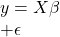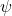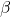# A note on double k-class estimators under elliptical symmetry

In this paper, estimation of the regression vector parameter in the multiple regression
modelis considered, when the error term belongs to the
class of elliptically contoured distributions (ECD), say,,
whereis unknown andis a symmetric p.d known matrix with the characteristic
generator. It is well-known that UMVU estimator ofhas the
form. In this paper using integral series representation of ECDs, the dominance conditions of double k-class estimators given byover UMVUE, have been derived under weighted quadratic loss function.

Fulltext